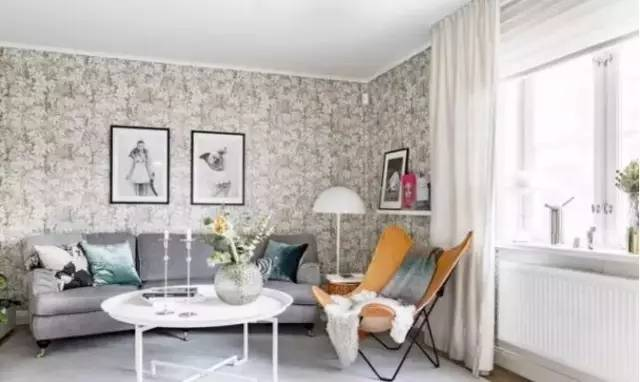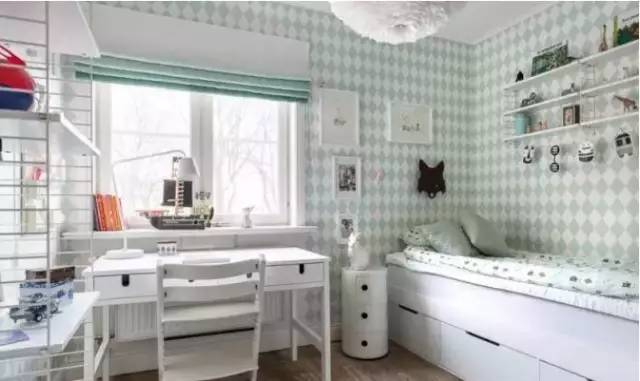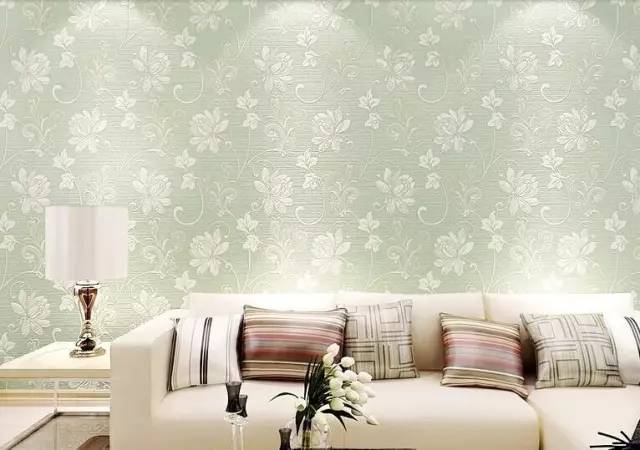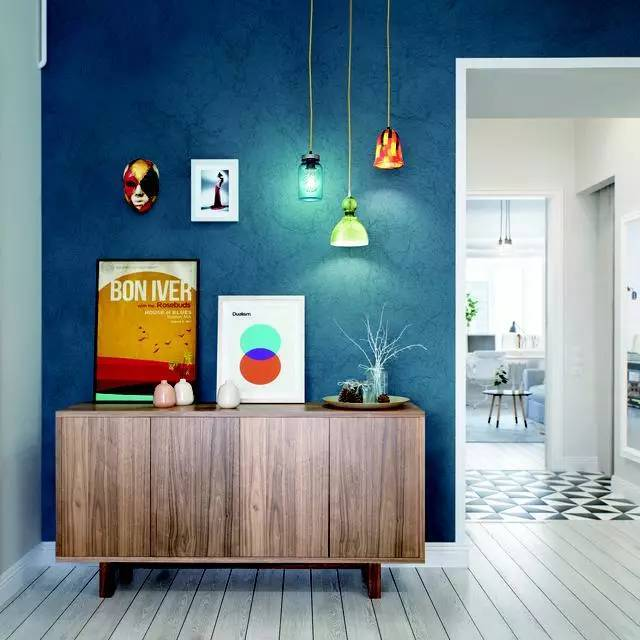|

# 墙纸鼓包、翘边、霉变、变色怎么办?这些方法可以帮到你!

墙纸固然能给房间增色不少，但是墙纸在铺贴后经常会出现一系列问题，比如翘边、开裂、霉变、变色等，非常让人闹心，那么墙纸为何会出现这些问题？出现问题后应该怎么处理呢？1 壁纸表面鼓包

分析：

（1）上胶不匀，局部缺胶液，壁纸干燥后形成气泡空鼓。

（2）局部胶液太多、太稠，无法完全自然吸收，壁纸干燥后形成硬块鼓包。

（3）基层腻子、涂料不牢固脱层，涂刷基膜未完全干燥，施工造成基层脱层，引起壁纸表面鼓包。

处理方法：安装前必须是基膜完全干后才安装壁纸，上胶不匀的空鼓可以用针筒注胶方式；胶液无法完全吸收的空鼓可用针筒注入稀释后的胶液软化胶块；基层脱层的轻微空鼓可采用注白乳胶方式修复，严重的只能撕揭壁纸处理好基层重新安装。2 壁纸受潮霉变现象

分析：霉菌孢子长时间在潮湿且一定温度的环境中，迅速的滋生蔓延所产生的变化，被称作霉变。一般霉变需要4个条件：霉菌源（空气中会携带霉菌孢子）、潮湿的环境、持续的较高温度、供霉菌孢子生长的营养基。

处理方法：

（1）做好墙体防水，另一侧墙为卫生间需格外注意。

（2）涂刷基膜要均匀，完全封闭住墙面。

（3）选用优质的墙纸胶，防止劣质的墙纸胶变质霉变。

（4）施工中墙纸胶要均匀适量，多余胶液要用刮板或其他专业工具刮出。

（5）墙纸施工后且接缝彻底干燥后（约48小时，具体依据现场环境和墙纸基材决定），开窗通风散发室内湿度。3 墙纸变色

分析：墙纸变色主要受阳光照射、氧化或受潮等原因。长时间阳光照射产生的色变一般会比较均匀，大多数不会影响到效果。氧化或受潮导致的墙纸变色会直接影响视觉效果，尤其带有金属印刷材料产品变色更加严重（如金、银色和金箔墙纸）。

处理方法：

（1）在施工过程中，杜绝外来的一切酸性和碱性物质的带入，以免污染墙纸。

（2）做好墙体防水，避免因湿度过大引起墙纸变色。

（3）墙面选用的腻子PH值为中性7±0.5，确保墙面已经彻底封闭处理好，隔绝从墙体中反渗出来碱性物质。

（4）选用PH值中性的优质基膜和墙纸胶，基膜涂刷一定要封闭住墙面，一定不能漏涂，墙纸胶均匀适量。

（5）酒精、杀虫剂等化学液体不要直接喷洒到墙纸表面。4 墙纸翘边、开缝

分析：构成墙纸翘边的因素较多，而且彼此间有着不同程度的关联，应综合多方面的信息，分析问题的实质，才能得出导致墙纸翘边、开缝的原因。

可能原因及处理方法：

1、施工不当：

（1）日光晒、劲风吹刮，引起收缩不均，局部开裂。

（2）软化时间过长，膨胀太长，干后形成缩缝。

（3）胶液浓度太低，粘力不足，不能粘住墙纸。

（4）局部胶液过多，收缩不均。

（5）粘贴时刮板用力过大，或施工时过多地拉长和拉宽壁纸，导致拉伸过量，干后形成缩缝。

（6）双层切割时刀口重复，显缝。

（7）操作者对缝不严。

处理方法：纯纸和PVC壁纸，用湿毛巾湿润接缝，过4-5分钟揭开壁纸，再刷胶重新再贴。无纺纸和丝绒纤维壁纸，没有膨胀性的壁纸，一般可以整张撕揭，先揭开壁纸，用拇指和食指轻轻依次拉长再刷胶粘贴。2、产品不当：包括产品的选用不当和产品的调配不当。施工前，应首先评估一下将要进行施工的墙纸，最好能事先做一下尝试性的粘贴试验，选择合适的胶粘产品，不能单靠经验行事。如用性能一般的墙纸胶粉粘贴厚重墙布；产品调配时没有依据包装说明；墙纸胶粉开胶时间过短，搅拌不均匀，未能完全糊化；开胶方法不当，造成抛团，胶浆粘度偏低等。3、环境因素：包括施工的温度与湿度条件、墙面基层（腻子）的结实度、墙面的光洁度与吸水性、墙面的封闭处理效果、施工表面的承载能力等，在这种情况下盲目施工，必然会出现问题。好的墙纸效果呈现还需要好的铺贴状态做保障，多一点用心，多一些省心！

`声明：本文由入驻焦点开放平台的作者撰写，除焦点官方账号外，观点仅代表作者本人，不代表焦点立场错误信息举报电话： 400-099-0099，邮箱：jubao@vip.sohu.com，或点此进行意见反馈，或点此进行举报投诉。`A B C D E F G H J K L M N P Q R S T W X Y Z
A - B - C - D - E
• A
• 鞍山
• 安庆
• 安阳
• 安顺
• 安康
• 澳门
• B
• 北京
• 保定
• 包头
• 巴彦淖尔
• 本溪
• 蚌埠
• 亳州
• 滨州
• 北海
• 百色
• 巴中
• 毕节
• 保山
• 宝鸡
• 白银
• 巴州
• C
• 承德
• 沧州
• 长治
• 赤峰
• 朝阳
• 长春
• 常州
• 滁州
• 池州
• 长沙
• 常德
• 郴州
• 潮州
• 崇左
• 重庆
• 成都
• 楚雄
• 昌都
• 慈溪
• 常熟
• D
• 大同
• 大连
• 丹东
• 大庆
• 东营
• 德州
• 东莞
• 德阳
• 达州
• 大理
• 德宏
• 定西
• 儋州
• 东平
• E
• 鄂尔多斯
• 鄂州
• 恩施
F - G - H - I - J
• F
• 抚顺
• 阜新
• 阜阳
• 福州
• 抚州
• 佛山
• 防城港
• G
• 赣州
• 广州
• 桂林
• 贵港
• 广元
• 广安
• 贵阳
• 固原
• H
• 邯郸
• 衡水
• 呼和浩特
• 呼伦贝尔
• 葫芦岛
• 哈尔滨
• 黑河
• 淮安
• 杭州
• 湖州
• 合肥
• 淮南
• 淮北
• 黄山
• 菏泽
• 鹤壁
• 黄石
• 黄冈
• 衡阳
• 怀化
• 惠州
• 河源
• 贺州
• 河池
• 海口
• 红河
• 汉中
• 海东
• 怀来
• I
• J
• 晋中
• 锦州
• 吉林
• 鸡西
• 佳木斯
• 嘉兴
• 金华
• 景德镇
• 九江
• 吉安
• 济南
• 济宁
• 焦作
• 荆门
• 荆州
• 江门
• 揭阳
• 金昌
• 酒泉
• 嘉峪关
K - L - M - N - P
• K
• 开封
• 昆明
• 昆山
• L
• 廊坊
• 临汾
• 辽阳
• 连云港
• 丽水
• 六安
• 龙岩
• 莱芜
• 临沂
• 聊城
• 洛阳
• 漯河
• 娄底
• 柳州
• 来宾
• 泸州
• 乐山
• 六盘水
• 丽江
• 临沧
• 拉萨
• 林芝
• 兰州
• 陇南
• M
• 牡丹江
• 马鞍山
• 茂名
• 梅州
• 绵阳
• 眉山
• N
• 南京
• 南通
• 宁波
• 南平
• 宁德
• 南昌
• 南阳
• 南宁
• 内江
• 南充
• P
• 盘锦
• 莆田
• 平顶山
• 濮阳
• 攀枝花
• 普洱
• 平凉
Q - R - S - T - W
• Q
• 秦皇岛
• 齐齐哈尔
• 衢州
• 泉州
• 青岛
• 清远
• 钦州
• 黔南
• 曲靖
• 庆阳
• R
• 日照
• 日喀则
• S
• 石家庄
• 沈阳
• 双鸭山
• 绥化
• 上海
• 苏州
• 宿迁
• 绍兴
• 宿州
• 三明
• 上饶
• 三门峡
• 商丘
• 十堰
• 随州
• 邵阳
• 韶关
• 深圳
• 汕头
• 汕尾
• 三亚
• 三沙
• 遂宁
• 山南
• 商洛
• 石嘴山
• T
• 天津
• 唐山
• 太原
• 通辽
• 铁岭
• 泰州
• 台州
• 铜陵
• 泰安
• 铜仁
• 铜川
• 天水
• 天门
• W
• 乌海
• 乌兰察布
• 无锡
• 温州
• 芜湖
• 潍坊
• 威海
• 武汉
• 梧州
• 渭南
• 武威
• 吴忠
• 乌鲁木齐
X - Y - Z
• X
• 邢台
• 徐州
• 宣城
• 厦门
• 新乡
• 许昌
• 信阳
• 襄阳
• 孝感
• 咸宁
• 湘潭
• 湘西
• 西双版纳
• 西安
• 咸阳
• 西宁
• 仙桃
• 西昌
• Y
• 运城
• 营口
• 盐城
• 扬州
• 鹰潭
• 宜春
• 烟台
• 宜昌
• 岳阳
• 益阳
• 永州
• 阳江
• 云浮
• 玉林
• 宜宾
• 雅安
• 玉溪
• 延安
• 榆林
• 银川
• Z
• 张家口
• 镇江
• 舟山
• 漳州
• 淄博
• 枣庄
• 郑州
• 周口
• 驻马店
• 株洲
• 张家界
• 珠海
• 湛江
• 肇庆
• 中山
• 自贡
• 资阳
• 遵义
• 昭通
• 张掖
• 中卫

1室1厅1厨1卫1阳台

1
2
3
4
5

0
1
2

1

1

0
1
2
3报名成功，资料已提交审核A B C D E F G H J K L M N P Q R S T W X Y Z
A - B - C - D - E
• A
• 鞍山
• 安庆
• 安阳
• 安顺
• 安康
• 澳门
• B
• 北京
• 保定
• 包头
• 巴彦淖尔
• 本溪
• 蚌埠
• 亳州
• 滨州
• 北海
• 百色
• 巴中
• 毕节
• 保山
• 宝鸡
• 白银
• 巴州
• C
• 承德
• 沧州
• 长治
• 赤峰
• 朝阳
• 长春
• 常州
• 滁州
• 池州
• 长沙
• 常德
• 郴州
• 潮州
• 崇左
• 重庆
• 成都
• 楚雄
• 昌都
• 慈溪
• 常熟
• D
• 大同
• 大连
• 丹东
• 大庆
• 东营
• 德州
• 东莞
• 德阳
• 达州
• 大理
• 德宏
• 定西
• 儋州
• 东平
• E
• 鄂尔多斯
• 鄂州
• 恩施
F - G - H - I - J
• F
• 抚顺
• 阜新
• 阜阳
• 福州
• 抚州
• 佛山
• 防城港
• G
• 赣州
• 广州
• 桂林
• 贵港
• 广元
• 广安
• 贵阳
• 固原
• H
• 邯郸
• 衡水
• 呼和浩特
• 呼伦贝尔
• 葫芦岛
• 哈尔滨
• 黑河
• 淮安
• 杭州
• 湖州
• 合肥
• 淮南
• 淮北
• 黄山
• 菏泽
• 鹤壁
• 黄石
• 黄冈
• 衡阳
• 怀化
• 惠州
• 河源
• 贺州
• 河池
• 海口
• 红河
• 汉中
• 海东
• 怀来
• I
• J
• 晋中
• 锦州
• 吉林
• 鸡西
• 佳木斯
• 嘉兴
• 金华
• 景德镇
• 九江
• 吉安
• 济南
• 济宁
• 焦作
• 荆门
• 荆州
• 江门
• 揭阳
• 金昌
• 酒泉
• 嘉峪关
K - L - M - N - P
• K
• 开封
• 昆明
• 昆山
• L
• 廊坊
• 临汾
• 辽阳
• 连云港
• 丽水
• 六安
• 龙岩
• 莱芜
• 临沂
• 聊城
• 洛阳
• 漯河
• 娄底
• 柳州
• 来宾
• 泸州
• 乐山
• 六盘水
• 丽江
• 临沧
• 拉萨
• 林芝
• 兰州
• 陇南
• M
• 牡丹江
• 马鞍山
• 茂名
• 梅州
• 绵阳
• 眉山
• N
• 南京
• 南通
• 宁波
• 南平
• 宁德
• 南昌
• 南阳
• 南宁
• 内江
• 南充
• P
• 盘锦
• 莆田
• 平顶山
• 濮阳
• 攀枝花
• 普洱
• 平凉
Q - R - S - T - W
• Q
• 秦皇岛
• 齐齐哈尔
• 衢州
• 泉州
• 青岛
• 清远
• 钦州
• 黔南
• 曲靖
• 庆阳
• R
• 日照
• 日喀则
• S
• 石家庄
• 沈阳
• 双鸭山
• 绥化
• 上海
• 苏州
• 宿迁
• 绍兴
• 宿州
• 三明
• 上饶
• 三门峡
• 商丘
• 十堰
• 随州
• 邵阳
• 韶关
• 深圳
• 汕头
• 汕尾
• 三亚
• 三沙
• 遂宁
• 山南
• 商洛
• 石嘴山
• T
• 天津
• 唐山
• 太原
• 通辽
• 铁岭
• 泰州
• 台州
• 铜陵
• 泰安
• 铜仁
• 铜川
• 天水
• 天门
• W
• 乌海
• 乌兰察布
• 无锡
• 温州
• 芜湖
• 潍坊
• 威海
• 武汉
• 梧州
• 渭南
• 武威
• 吴忠
• 乌鲁木齐
X - Y - Z
• X
• 邢台
• 徐州
• 宣城
• 厦门
• 新乡
• 许昌
• 信阳
• 襄阳
• 孝感
• 咸宁
• 湘潭
• 湘西
• 西双版纳
• 西安
• 咸阳
• 西宁
• 仙桃
• 西昌
• Y
• 运城
• 营口
• 盐城
• 扬州
• 鹰潭
• 宜春
• 烟台
• 宜昌
• 岳阳
• 益阳
• 永州
• 阳江
• 云浮
• 玉林
• 宜宾
• 雅安
• 玉溪
• 延安
• 榆林
• 银川
• Z
• 张家口
• 镇江
• 舟山
• 漳州
• 淄博
• 枣庄
• 郑州
• 周口
• 驻马店
• 株洲
• 张家界
• 珠海
• 湛江
• 肇庆
• 中山
• 自贡
• 资阳
• 遵义
• 昭通
• 张掖
• 中卫• 手机• 分享
• 设计
免费设计
• 计算器
装修计算器
• 入驻
合作入驻
• 联系
联系我们
• 置顶
返回顶部